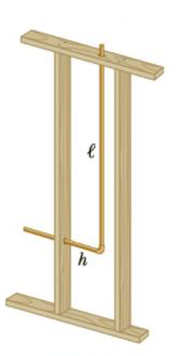Chapter 10, Problem 51AP

Chapter
Section
Textbook Problem

Inside the wall of a house, an L-shaped section of hot-water pipe consists of three parts: a straight horizontal piece h = 28.0 cm long, an elbow, and a straight, vertical piece ℓ = 134 cm long (Fig. P10.51). A stud and a second- story floorboard hold the ends of this section of copper pipe stationary. Find the magnitude and direction of the displacement of the pipe elbow when the water flow is turned on, raising the temperature of the pipe from 18.0°C to 46.5°C.Figure P10.51

To determine
The magnitude and direction of displacement of the pipe.

Explanation

Section 1:

To determine: The magnitude of displacement of the pipe.

Answer: The magnitude of displacement of the pipe is 0.66 mm.

Explanation:

Given info: Length of the straight horizontal piece is h=28.0cm . Length of the vertical piece is l=134cm. The temperature is raised from T1=18οC to T2=46.5οC . The co-efficient of linear expansion ( α ) of copper is 17×106/οC .

Formula to calculate the expansion along the horizontal direction is,

ΔLh=αh(T2T1) (I)

Formula to calculate the expansion along the vertical direction is,

ΔLv=αl(T2T1) (II)

Formula to calculate the total expansion is,

ΔL=(ΔLh)2+(ΔLv)2 (III)

Substitute Equations (I) and (II) in (III).

ΔL=[αh(T2T1)]2+[αl(T2T1)]2=α(T2T1)h2+l2

Substitute 17×106/οC for α , 18οC for T1 , 46.5οC for T2 , 28.0 cm for h and 134 cm for l in the above equation to get ΔL .

ΔL=(17×106/οC)[(46.5οC)(18οC)](28.0cm)2+(134cm)2=(17×106/οC)(28

Still sussing out bartleby?

Check out a sample textbook solution.

See a sample solution

The Solution to Your Study Problems

Bartleby provides explanations to thousands of textbook problems written by our experts, many with advanced degrees!

Get Started

The thermic effect of food plays a major role in energy expenditure, T F

Nutrition: Concepts and Controversies - Standalone book (MindTap Course List)

___ is the actual path length. (2.2)

An Introduction to Physical Science

14-46 Write the common name for each thiol in Problem 14-45.

Introduction to General, Organic and Biochemistry

What does the acronym MUFA stand for?

Organic And Biological Chemistry